# Angle side angle

Angle side angle

Have you ever observed that two copies of a single photograph of the same size are identical?Similarly, ATM cards issued by the same bank are identical.Such figures are called congruent figures.

You may have noticed an ice tray in your refrigerator.

The molds inside the tray that are used for making ice are congruent.Have you ever struggled to place a new ink chamber in a pen?

This could have happened because the new ink chamber is not the same size as the one you want to replace.

Remember that whenever identical objects are to be produced, the concept of congruence is taken into consideration in making the cast.

In this section, we will explore the ASA congruence theorem using real-life examples.

Check out the interactive simulation to know more about the lesson and try your hand at solving a few interesting practice questions on this theorem at the end of the page.

## Lesson Plan

 1 What Is the Definition of Angle Side Angle? 2 Thinking out of the Box! 3 Solved Examples on Angle Side Angle 4 Challenging Questions on Angle Side Angle 5 Interactive Questions on Angle Side Angle

## What Is the Definition of Angle Side Angle?

If two triangles are congruent by the angle side angle formula, it means that:

• Three sides of one triangle will be (respectively) equal to the three sides of the other.
• Three angles of one triangle will be (respectively) equal to the three angles of the other.

However, in order to be sure that two triangles are congruent, we do not necessarily need to have information about all sides and all angles.

There are five criteria to find if two triangles are congruent:

1. SSS (side, side, side )
2. SAS (side, angle, side )
3. ASA (side, angle, side)
4. AAS (angle, angle, side), and
5. HL (hypotenuse, leg)

In this chapter, we will study the ASA (Angle-Side-Angle) postulate, angle side angle calculator, and angle side angle examples.
Angle Side Angle Definition

It states that if two angles of one triangle, and the side between these two angles, are respectively equal to the two angles and the side between the angles of another triangle, then the two triangles will be congruent to each other by ASA rule.

Let us understand this through a diagram.

Consider the following two triangles, $$\Delta ABC$$ and $$\Delta DEF$$: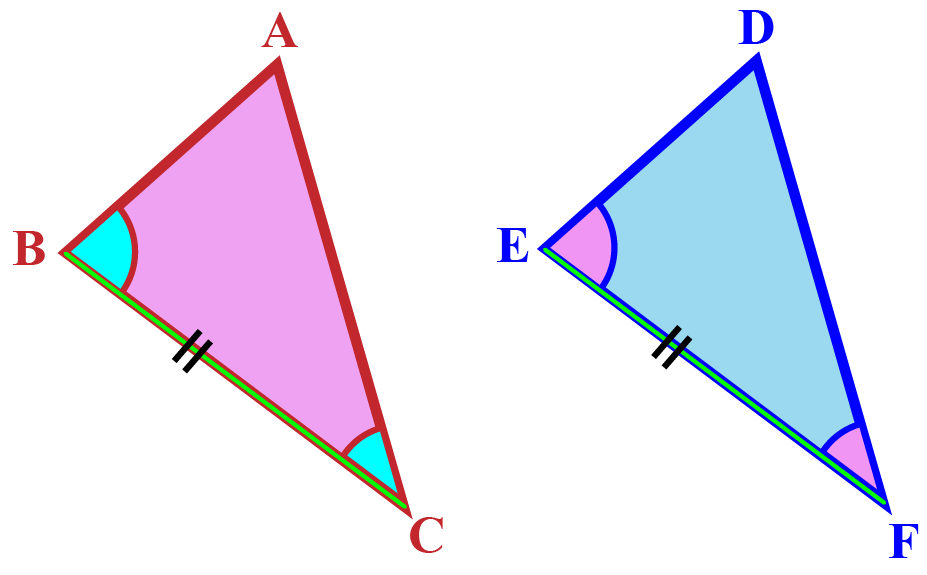We are given that,

$\begin{gathered} BC = EF \hfill \\ \angle B = \angle E \hfill \\ \angle C = \angle F \hfill \\ \end{gathered}$

We say that by ASA criterion:

 $$\Delta ABC \cong \Delta DEF$$

## What Are Congruent Triangles?

If two triangles are congruent it means that:

Three sides of one triangle will be (respectively) equal to the three sides of the other.

Three angles of one triangle will be (respectively) equal to the three angles of the other.

These triangles should superimpose each other completely by side to side and angle to angle.

## What Is Meant by the ASA Congruence theorem?

Angle side angle theorem states that two triangles are congruent if two angles and the included side of one triangle are equal to two angles and the included side of the other triangle

Proof:

Consider the following two triangles, $$\Delta ABC$$ and $$\Delta DEF$$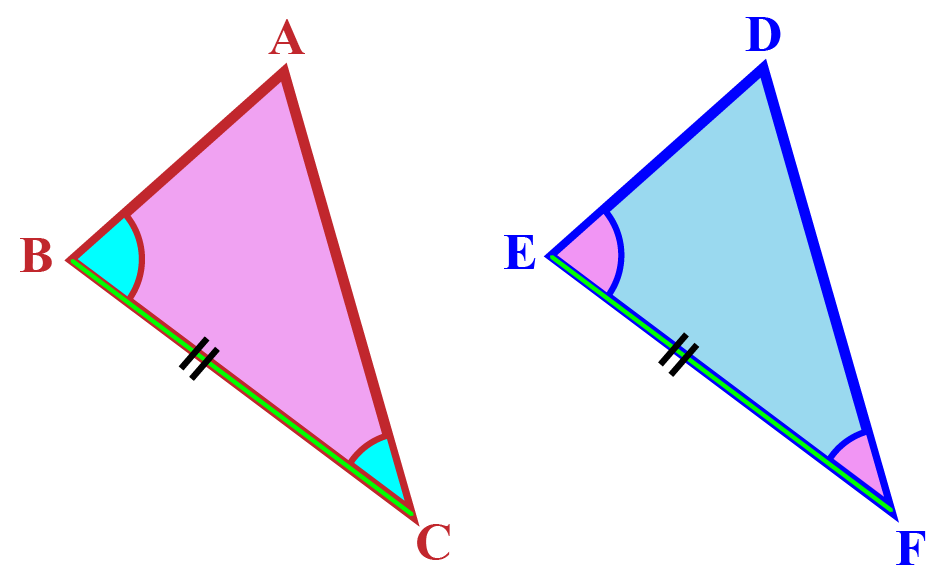We are given that,

$\begin{gathered} BC = EF \hfill \\ \angle B = \angle E \hfill \\ \angle C = \angle F \hfill \\ \end{gathered}$

Can we say that $$\Delta ABC$$ and $$\Delta DEF$$ are congruent?

Let us first do a thought experiment and try to superimpose $$\Delta DEF$$ on $$\Delta ABC$$.

Align $$EF$$ exactly with $$BC$$.

Since $$\angle B = \angle E$$, the direction of $$ED$$ will be the same as the direction of $$BA$$.

Similarly, since $$\angle C = \angle F$$, the direction of $$FD$$ will be the same as the direction of $$CA$$.

This means that the point of intersection of $$ED$$ and $$FD$$ (which is $$D$$) will coincide exactly with the point of intersection of $$BA$$ and $$CA$$ (which is $$A$$).

Thus, since all the three vertices of the two triangles (can be made to) respectively coincide, the two triangles are congruent by angle side angle triangle congruence theorem.

 $$\Delta ABC \cong \Delta DEF$$Think Tank
• Can you explain the five ways to prove that triangles are congruent?
• Does the diagonal of a rectangle divide the rectangle into two equal triangles?

## Solved Examples

 Example 1

Parallelogram ABCD is made up of two triangles $$\Delta ABC$$ and $$\Delta ACD$$. It is given that  $$\angle \text{ ABC is } 70^\circ$$ and $$\angle \text{ BCA is } 30^\circ$$, which are equal to $$\angle \text{ CDA}$$ and  $$\angle \text{ DAC}$$ respectively. Side BC is equal to side AD. Can you tell which property is used to tell whether $$\Delta ABC$$ and $$\Delta ACD$$ are congurent?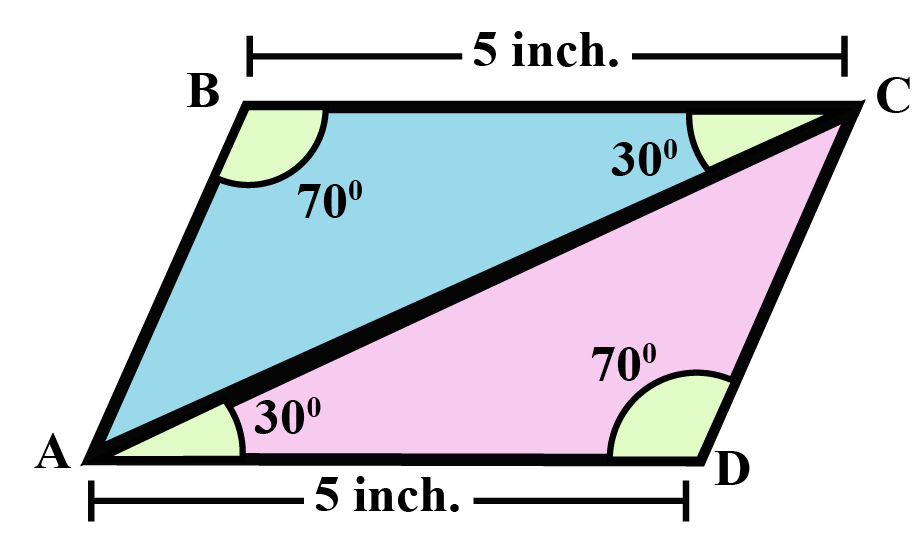Solution

Given,

$$\angle \text{ ABC} = \angle \text{ CDA} = 70^\circ$$
$$\angle \text{ BCA} = \angle \text{ DAC} = 30^\circ$$
Side BC = Side AD.
$$\therefore$$    By ASA criterion,

 $$\Delta ABC \cong \Delta ACD$$
 Example 2

Sean wants to find the value of 'x' in $$\angle ADC$$. It is given that $$\Delta ABC \cong \Delta ACD$$ by ASA criterion. Also, find the total measure of $$\angle ADC$$?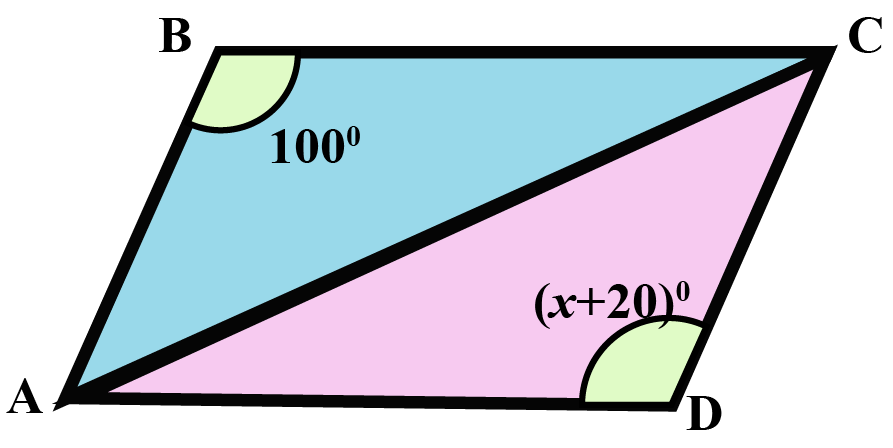Solution

In the given figure, $$\Delta ABC \cong \Delta ACD$$ ........................by ASA property

(I)  $$\angle ABC$$ = $$\angle ADC$$

\begin{align*} 100^\circ &= (x+20)^\circ \\ x^\circ &= 100^\circ - 20^\circ \\ x^\circ &= 80^\circ\\ \end{align*}

(ii) The total measure of $$\angle ADC$$:\begin{align*}\angle ADC &= (x+20)^\circ \\ \text{ We know } \ \ x&=80^\circ \\ \therefore \angle ADC &= 80^\circ+20^\circ=100^\circ \end{align*}

 $$\therefore$$ $$x^\circ = 80^\circ$$ and $$\angle ADC =100^\circ$$
 Example 3

In the given figure, there are two triangles, QPS and QRS, having side PQ and side QR equal to each other. Can you find out whether $$\Delta PQS \cong \Delta RQS$$?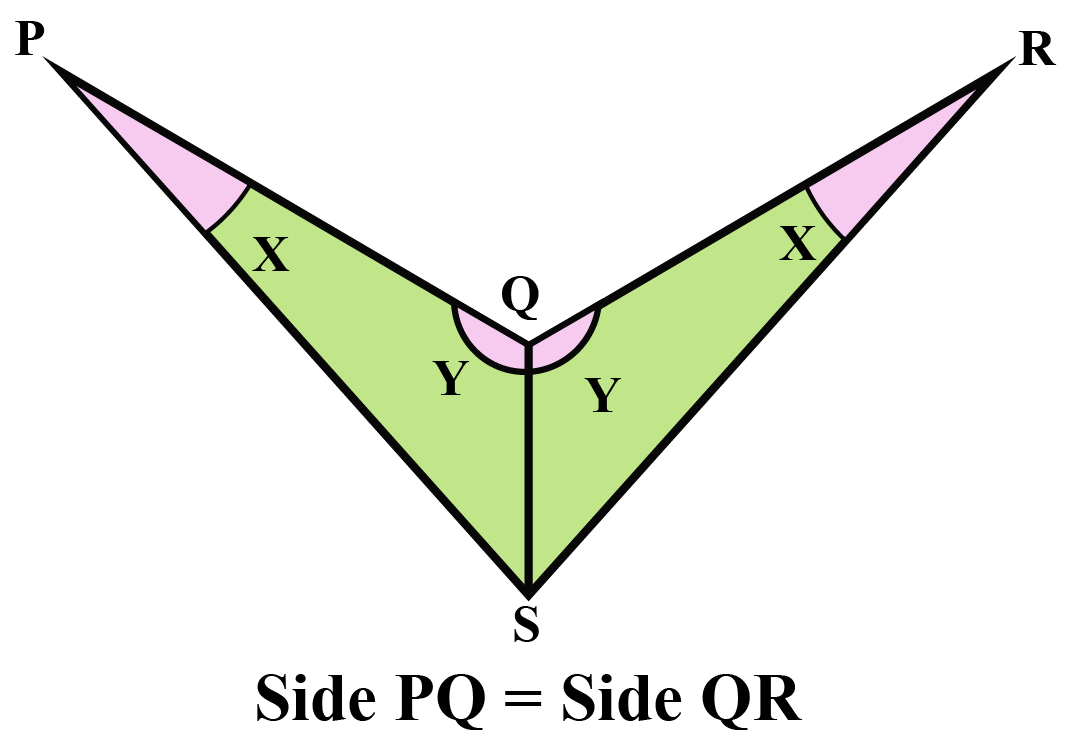Solution

Given,

\begin{align*} \angle SPQ &= \angle SRQ \\ \angle PQS &= \angle RQS \\ \text {also, Side PQ} &= \text {Side QR} \end{align*}

We have two angles and one side common in both triangles.

$$\therefore$$ By using ASA criterion,

 $$\therefore \Delta PQS \cong \Delta RQS$$Challenging Questions
• What is (side, side, side) SSS and SAS (side, angle, side ) postulate?
• Can you give an example of AAS (angle, angle, side), and HL (hypotenuse, leg) congruency?
• By using SAS congruency, prove that angles opposite to the equal side of an isosceles triangle are equal.

## Interactive Questions

Here are a few activities for you to practice. Select/Type your answer and click the "Check Answer" button to see the result.

## Let's Summarize

This mini-lesson targeted the fascinating concept of an angle side angle criterion. The math journey around the angle side angle criterion starts with what a student already knows, and goes on to creatively crafting a fresh concept in the young minds. Done in a way that is not only relatable and easy to grasp, but will also stay with them forever. Here lies the magic with Cuemath.

## About Cuemath

At Cuemath, our team of math experts is dedicated to making learning fun for our favorite readers, the students!

Through an interactive and engaging learning-teaching-learning approach, the teachers explore all angles of a topic.

Be it worksheets, online classes, doubt sessions, or any other form of relation, it’s the logical thinking and smart learning approach that we, at Cuemath, believe in.

## 1. How do you solve ASA?

To solve the ASA criterion, we find out the two equal angles and the common side between them.

And by using congruency ratios, we find out the unknown sides or angles.

## 2. What is the ASA theorem?

ASA congruence criterion states that if two angles of one triangle, and the side contained between these two angles, are respectively equal to two angles of another triangle and the side contained between them, then the two triangles will be congruent.

## 3. How do you make an ASA triangle?

ASA congruence criterion states that if two angles of one triangle, and the side contained between these two angles, are respectively equal to two angles of another triangle and the side contained between them, then the two triangles will be congruent.

To make an ASA triangle, we find out the two equal angles and the common side between them.

## 4. How do you find the Angle Angle Side?

In angle-angle side(AAS) if two angles and the one non-included side of one triangle are congruent to two angles and the non-included side of another triangle, then these two triangles are congruent.

## 5. Is Angle Side Side a theorem?

No, Angle Side Side is not a theorem

## 6. How do I know my SSS, SAS, ASA, and AAS?

The full form of the given terms are:
SSS (side, side, side), SAS (side, angle, side), ASA (angle, side, angle), and AAS (angle, angle, side).

## 7. Can you solve a triangle with 3 angles?

A triangle with 3 angles is impossible to solve further since there is no side included.

Download Triangles and Quadrilaterals Worksheets
Triangles and Quadrilaterals
grade 9 | Questions Set 1
Triangles and Quadrilaterals
grade 9 | Answers Set 1
Triangles and Quadrilaterals
grade 9 | Questions Set 2
Triangles and Quadrilaterals
grade 9 | Answers Set 2
More Important Topics
Numbers
Algebra
Geometry
Measurement
Money
Data
Trigonometry
Calculus